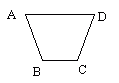Name: ___________________Date:___________________

 Email us to get an instant 20% discount on highly effective K-12 Math & English kwizNET Programs!

### MEAP Preparation - Grade 5 Mathematics3.5 Polygons

 Polygons are closed figures that are formed by line segments. Each side is a line segment. The point where two line segments meet is a vertex. The sides do not cross each other and exactly two sides meet at every vertex. The segments that form the boundary are sides, which meet at a vertices to form the interior angle. If we connect two vertices that are not adjacent on the polygon, we form a diagonal. Examples: Squares, Triangles, Rectangles, etc. Directions: Answer the following questions. Also draw 10 examples of polygons an name the sides, vertices and diagonals on a sheet of paper.
 Q 1: Polygons are named by starting at one vertex and naming all the other vertices in order. Name this figure.Polygon ABCDPolygon ACDBPolygon ADBC Q 2: In polygons, the point where two line segments meet is called avertexfaceside Q 3: Which of these is not a polygon?squarerectangletrianglecircle Q 4: Which of the following figures is not a polygon?DCAB Q 5: In a polygon each side is aline segmentpointdot Q 6: Which of the following is not a closed figure?CBAD Question 7: This question is available to subscribers only! Question 8: This question is available to subscribers only!